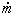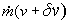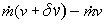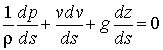### Euler's Equation of Motion

Home -> Lecture Notes -> Fluid Mechanics -> Unit-IIMass in per unit time = rAv =For steady flow, mass out per unit time =Rate of momentum in =Rate of momentum out =Rate of increase of momentum from AB to CD == rAv dv à 1

Force due to p in the direction of motion = pA

Force due to p + dp opposing the direction of motion = (p + dp)(A + dA)

Force due to pside producing a component in the direction of motion = psidedA

Force due to mg producing a component opposing the direction of motion = mgcos(q)

Resultant force in the direction of motion = pA - (p + dp)(A + dA) + psidedA - mgcos(q) à 2

The value of pside will vary from p at AB to p + dp at CD, and can be taken as p + kdp where k is fraction.

Mass of fluid element ABCD = m = rg(A + 1/2 dA) ds

And ds = dz/cos(q); since cos(q) = dz/ds

Substituting in equn.2,

Resultant force in the direction of motion = pA - (p + dp)(A + dA) + p + kdp - rg(A + 1/2 dA) dz

Neglecting products of small quantities,

Resultant force in the direction of motion = -Adp - rgAdz à 3

Applying Newton's second law, (i.e., equating equns.1 & 3)or, in the limit as ds à 0,This is known as Euler's equation, giving, in differential formthe relationship between p, v, r and elevation z, along a streamline for steady flow.

It can not be integrated until the relationship density and pressure is known.

For incompressible fluid, r is constant; therefore the Euler's equation is integrated to give the following:which is nothing but the Bernoulli equation.